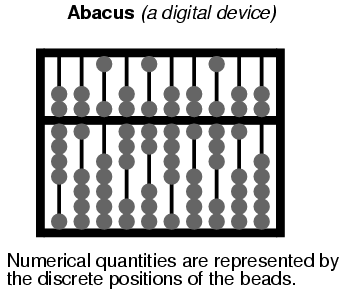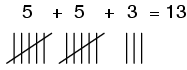# 1.1: Numbers and Symbols

First, we have to distinguish the difference between numbers and the symbols we use to represent numbers. A number is a mathematical quantity, usually correlated in electronics to a physical quantity such as voltage, current, or resistance. There are many different types of numbers. Here are just a few types, for example:Different types of numbers find different application in the physical world. Whole numbers work well for counting discrete objects, such as the number of resistors in a circuit. Integers are needed when negative equivalents of whole numbers are required. Irrational numbers are numbers that cannot be exactly expressed as the ratio of two integers, and the ratio of a perfect circle’s circumference to its diameter (π) is a good physical example of this. The non-integer quantities of voltage, current, and resistance that we’re used to dealing with in DC circuits can be expressed as real numbers, in either fractional or decimal form. For AC circuit analysis, however, real numbers fail to capture the dual essence of magnitude and phase angle, and so we turn to the use of complex numbers in either rectangular or polar form.

If we are to use numbers to understand processes in the physical world, make scientific predictions, or balance our checkbooks, we must have a way of symbolically denoting them. In other words, we may know how much money we have in our checking account, but to keep record of it we need to have some system worked out to symbolize that quantity on paper, or in some other kind of form for record-keeping and tracking. There are two basic ways we can do this: analog and digital. With analog representation, the quantity is symbolized in a way that is infinitely divisible. With digital representation, the quantity is symbolized in a way that is discretely packaged.

You’re probably already familiar with an analog representation of money, and didn’t realize it for what it was. Have you ever seen a fund-raising poster made with a picture of a thermometer on it, where the height of the red column indicated the amount of money collected for the cause? The more money collected, the taller the column of red ink on the poster.This is an example of an analog representation of a number. There is no real limit to how finely divided the height of that column can be made to symbolize the amount of money in the account. Changing the height of that column is something that can be done without changing the essential nature of what it is. Length is a physical quantity that can be divided as small as you would like, with no practical limit. The slide rule is a mechanical device that uses the very same physical quantity—length—to represent numbers, and to help perform arithmetical operations with two or more numbers at a time. It, too, is an analog device.

On the other hand, a digital representation of that same monetary figure, written with standard symbols (sometimes called ciphers), looks like this:Unlike the “thermometer” poster with its red column, those symbolic characters above cannot be finely divided: that particular combination of ciphers stand for one quantity and one quantity only. If more money is added to the account (+ $40.12), different symbols must be used to represent the new balance ($35,995.50), or at least the same symbols arranged in different patterns. This is an example of digital representation. The counterpart to the slide rule (analog) is also a digital device: the abacus, with beads that are moved back and forth on rods to symbolize numerical quantities:Let’s contrast these two methods of numerical representation:Interpretation of numerical symbols is something we tend to take for granted, because it has been taught to us for many years. However, if you were to try to communicate a quantity of something to a person ignorant of decimal numerals, that person could still understand the simple thermometer chart!

The infinitely divisible vs. discrete and precision comparisons are really flip-sides of the same coin. The fact that digital representation is composed of individual, discrete symbols (decimal digits and abacus beads) necessarily means that it will be able to symbolize quantities in precise steps. On the other hand, an analog representation (such as a slide rule’s length) is not composed of individual steps, but rather a continuous range of motion. The ability for a slide rule to characterize a numerical quantity to infinite resolution is a trade-off for imprecision. If a slide rule is bumped, an error will be introduced into the representation of the number that was “entered” into it. However, an abacus must be bumped much harder before its beads are completely dislodged from their places (sufficient to represent a different number).

Please don’t misunderstand this difference in precision by thinking that digital representation is necessarily more accurate than analog. Just because a clock is digital doesn’t mean that it will always read time more accurately than an analog clock, it just means that the interpretation of its display is less ambiguous.

Divisibility of analog versus digital representation can be further illuminated by talking about the representation of irrational numbers. Numbers such as π are called irrational, because they cannot be exactly expressed as the fraction of integers, or whole numbers. Although you might have learned in the past that the fraction 22/7 can be used for π in calculations, this is just an approximation. The actual number “pi” cannot be exactly expressed by any finite, or limited, number of decimal places. The digits of π go on forever:It is possible, at least theoretically, to set a slide rule (or even a thermometer column) so as to perfectly represent the number π, because analog symbols have no minimum limit to the degree that they can be increased or decreased. If my slide rule shows a figure of 3.141593 instead of 3.141592654, I can bump the slide just a bit more (or less) to get it closer yet. However, with digital representation, such as with an abacus, I would need additional rods (place holders, or digits) to represent π to further degrees of precision. An abacus with 10 rods simply cannot represent any more than 10 digits worth of the number π, no matter how I set the beads. To perfectly represent π, an abacus would have to have an infinite number of beads and rods! The tradeoff, of course, is the practical limitation to adjusting, and reading, analog symbols. Practically speaking, one cannot read a slide rule’s scale to the 10th digit of precision, because the marks on the scale are too coarse and human vision is too limited. An abacus, on the other hand, can be set and read with no interpretational errors at all.

Furthermore, analog symbols require some kind of standard by which they can be compared for precise interpretation. Slide rules have markings printed along the length of the slides to translate length into standard quantities. Even the thermometer chart has numerals written along its height to show how much money (in dollars) the red column represents for any given amount of height. Imagine if we all tried to communicate simple numbers to each other by spacing our hands apart varying distances. The number 1 might be signified by holding our hands 1 inch apart, the number 2 with 2 inches, and so on. If someone held their hands 17 inches apart to represent the number 17, would everyone around them be able to immediately and accurately interpret that distance as 17? Probably not. Some would guess short (15 or 16) and some would guess long (18 or 19). Of course, fishermen who brag about their catches don’t mind overestimations in quantity!

Perhaps this is why people have generally settled upon digital symbols for representing numbers, especially whole numbers and integers, which find the most application in everyday life. Using the fingers on our hands, we have a ready means of symbolizing integers from 0 to 10. We can make hash marks on paper, wood, or stone to represent the same quantities quite easily:For large numbers, though, the “hash mark” numeration system is too inefficient.